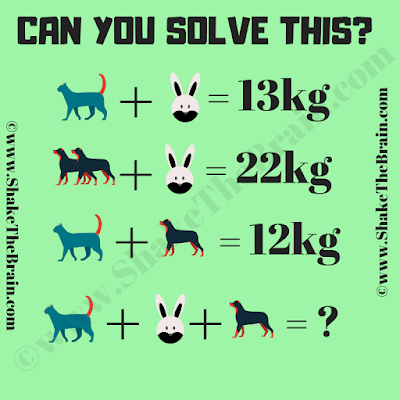This is an interesting math picture puzzle for school going students. Solving this math puzzle will help you to improve your mathematical skills. In this math puzzle, you are shown some mathematical equations. Your challenge is to solve these math equations and find the values of the given animals. Once you are able to find the value of each animal, solve the last equation to find the missing sum.Can you find the value of the missing sum?
Answer of this "Math Picture Puzzle", can be viewed by clicking on the answer button.